# Math Help: Formulas It Helps to Remember

09/21/2015

## Remember These Great Math Tips

Here are a few mathematical formulas that come in handy when you have them memorized.

### Quadratic Formula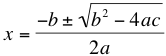Solve any quadratic equation in the form ax^2+bx+c=0 with this formula. For those formulas that are difficult to find the difference of squares, or if you are in a rush on an exam, the quadratic formula will be very nice to know.

### Statistical Combinations

To find the total possible different combinations of different elements, simply multiply them all together. For example, if there are 4 appetizers, 5 entrees, and 3 desserts on a menu, there are 4*5*3=60 different possible combinations.

### Circle Formulas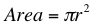The area of a circle is the square of the radius times π.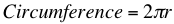Multiply π times the diameter, or twice the radius, of a circle. When working with arcs, just remember that an arc is a percentage of a circle. Since there are 360 degrees in a circle, first calculate the percentage of the whole circle the arc represents. If the arc is 90 degrees, it is 90/360=1/4 or 25%. Multiply the area or circumference of the whole circle by this percentage to find the area or circumference of the arc.

### Distance Formula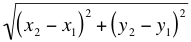If you know your Pythagorean theorem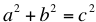, this equation will be intuitive. To determine the distance between two points, you can make a right triangle from them. square each side and then take the square root of the sum to obtain the length of the hypotenuse.

## For Tutoring in Sammamish

If you would like additional help with math, contact The Tutoring Center in Sammamish. We offer one-to-one tutoring programs in math, reading, writing, and test preparation to students of all ages and levels. Find out more about us at our webpage and call 425-202-7306 to schedule your free diagnostic assessment today.

### FREE CONSULTATION

Schedule your Free Diagnostic Assessment Today!
Learn more about
THE TUTORING CENTER
on the national website: